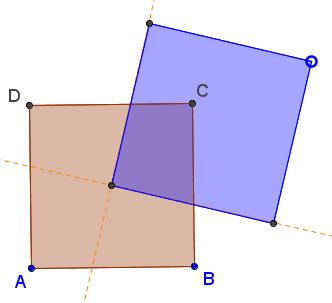Area of the Union of Two Squares

Squares ABCD and MNOP are congruent with side length 10, and O is the center of ABCD. What is the area of the region covered by the union of these squares [First Steps, p. 81].

3 November 2016, Created with GeoGebra

Solution

References

1. J. D. Faires, First Steps For Math Olympians, MAA, 2006The main point to observe is that the area of the intersection of the two squares is exactly 1/4 that of ABCD. In fact (and hopefully the applet helps with this realization), the area of the intersection and even its shape does not depend on the size of the second (MNOP) square as long as it is big enough to include a corner of ABCD. The extended side lines ON and OP of MNOP cut ABCD into four equal regions the same for all M on a fixed line through O.

The area covered by the union of the two squares is the sum of their areas minus the area of the intersection:

102 + 102 - 102/4 = 175.

In a more general case where the side length of MNOP is a, with a greater than the half diagonal of ABCD, the area of the union is given by

102 + a2 - 102/4.

The book offers a different proof based on the following diagram:where, after interchanging two equal right triangles, the intersection of the squares transforms into a clear quarter of ABCD.

(In a different vein: could you evaluate the difference of the remaining portions of the two squares without finding the area of their intersection? It is done for circles!)

In [Trigg, #121] the same problem is given with an extra condition that one of the squares divides a side of the other in a ratio 1:2, which is of cause a red herring.

References

1. C. W. Trigg, Mathematical Quickies, Dover, 1985, #12What Is Red Herring